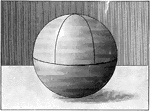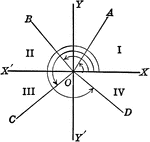Axes showing trigonometric angles, and quadrants.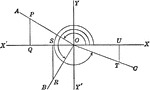### Reference Angles/Triangles Formed by Angles in Quadrants

Lines drawn to horizontal to form triangle ratios (reference triangles, angles).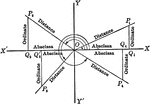### Reference Angles/Triangles Formed by Angles in Quadrants With Labels

Lines drawn to horizontal to form triangle ratios (reference triangles, angles). Axes, quadrants, abscissa,…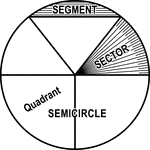### Circle with Segment, Semicircle, Sector, Quadrant, and Central Angle

Illustrations of a circle with segment, semicircle, sector, quadrant, and central angles drawn.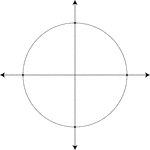### Unit Circle

Illustration of a unit circle (circle with a radius of 1) superimposed on the coordinate plane. The…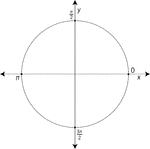### Unit Circle Labeled At Quadrantal Angles

Illustration of a unit circle (circle with a radius of 1) superimposed on the coordinate plane with…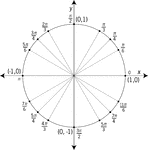### Unit Circle Labeled At Special Angles

Illustration of a unit circle (circle with a radius of 1) superimposed on the coordinate plane with…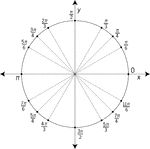### Unit Circle Labeled At Special Angles

Illustration of a unit circle (circle with a radius of 1) superimposed on the coordinate plane with…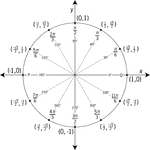### Unit Circle Labeled In 30° Increments With Values

Illustration of a unit circle (circle with a radius of 1) superimposed on the coordinate plane with…### Unit Circle Labeled In 45 ° Increments

Illustration of a unit circle (circle with a radius of 1) superimposed on the coordinate plane with…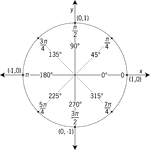### Unit Circle Labeled In 45° Increments With Values

Illustration of a unit circle (circle with a radius of 1) superimposed on the coordinate plane with…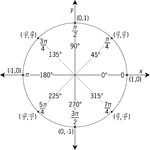### Unit Circle Labeled In 45° Increments With Values

Illustration of a unit circle (circle with a radius of 1) superimposed on the coordinate plane with…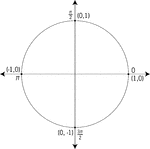### Unit Circle Labeled With Quadrantal Angles And Values

Illustration of a unit circle (circle with a radius of 1) superimposed on the coordinate plane. All…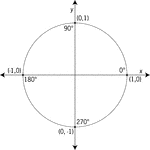### Unit Circle Labeled With Quadrantal Angles And Values

Illustration of a unit circle (circle with a radius of 1) superimposed on the coordinate plane with…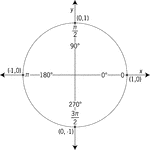### Unit Circle Labeled With Quadrantal Angles And Values

Illustration of a unit circle (circle with a radius of 1) superimposed on the coordinate plane with…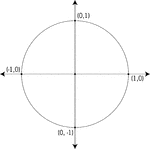### Unit Circle Labeled With Quadrantal Values

Illustration of a unit circle (circle with a radius of 1) superimposed on the coordinate plane. At each…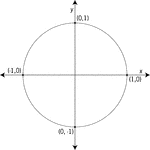### Unit Circle Labeled With Quadrantal Values

Illustration of a unit circle (circle with a radius of 1) superimposed on the coordinate plane with…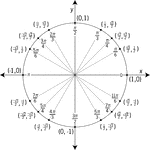### Unit Circle Labeled With Special Angles And Values

Illustration of a unit circle (circle with a radius of 1) superimposed on the coordinate plane with…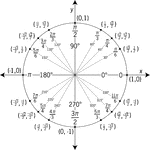### Unit Circle Labeled With Special Angles And Values

Illustration of a unit circle (circle with a radius of 1) superimposed on the coordinate plane with…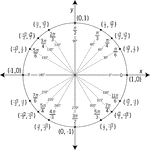### Unit Circle Labeled With Special Angles And Values

Illustration of a unit circle (circle with a radius of 1) superimposed on the coordinate plane with…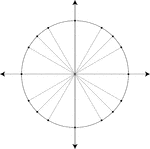### Unit Circle Marked At Special Angles

Illustration of a unit circle (circle with a radius of 1) superimposed on the coordinate plane. All…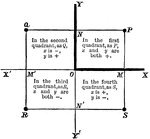### Coordinate Plane

A coordinate planes with the quadrants labeled showing which value (x or y) is positive or negative.### Sphere With Quadrants and Poles

A sphere with quadrants and poles. "A point on the surface of a sphere, which is at the distance of…